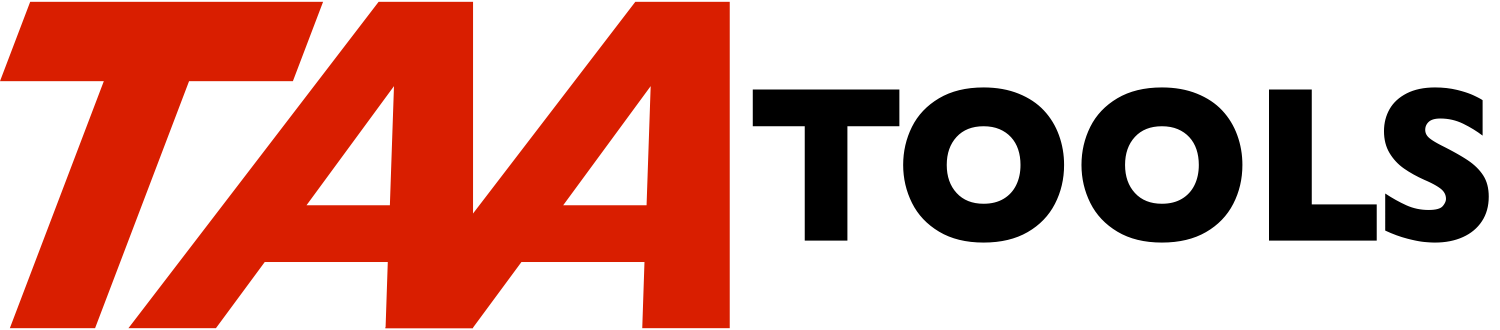`DIVIDE          DIVIDE                                 TAACLRP`
```
The Divide  command provides  a quotient,  a remainder,  and a  decimal
fraction  after dividing  a dividend  by a  divisor.   The  CL division
function  does not  provide for  a remainder nor  for a  fraction.  The
input variables must be specified as *DEC LEN(10 0).

A typical series of commands would be:

DCL        &DIVIDEND *DEC LEN(10 0)
DCL        &DIVISOR *DEC LEN(10 0)
DCL        "IENT *DEC LEN(10 0)
DCL        &REMAINDER *DEC LEN(10 0)
DCL        &DECFRACT *DEC LEN(9 9)
.
DIVIDE     DIVIDEND(&DIVIDEND) DIVISOR(&DIVISOR) +
QUOTIENT("IENT) REMAINDER(&REMAINDER)
DECFRACT(&DECFRACT)

If the dividend  is 333 and  the divisor is 12,  the quotient would  be
27 with a remainder of 9 and a fraction of .75.

If you  have dividend such  as 100.15, you  must convert it to  a whole
number  using multiplication or the TAA  MOVTODEC command.  The results
after the divide must then  be converted to provide the proper  decimal
positions.

Internally the C 'div' run time function is used.

DIVIDE escape messages you can monitor for
------------------------------------------

CPF9898    Divisor of 0

Escape messages from based on functions will be re-sent.

DIVIDE Command parameters                             *CMD
-------------------------

DIVIDEND      The  dividend  to be  divided  by  the divisor.    The
value must be specified as *DEC LEN(10 0).

DIVISOR       The  divisor  to divide  into  the dividend.    If the
divisor is 0,  CPF9898 is sent  as an escape  message.
The value must be specified as *DEC LEN(10 0).

QUOTIENT      The result of the  divide as a whole number.   This is
a  return  variable that  must  be  specified as  *DEC
LEN(10 0).

REMAINDER     The  remainder of the divide as  a whole number.  This
is a return  variable that must  be specified as  *DEC
LEN(10 0).

DECFRACT      The  decimal fraction  of the  whole number  after the
divide.    This  is a  return  variable  that  must be
specified as *DEC LEN(9 9).

Restrictions
------------

Because the command  returns variables, DIVIDE  may only be  used in  a
CL program.

Prerequisites
-------------

The following TAA Tools must be on your system:

SNDESCINF       Send escape information
SNDESCMSG       Send escape message

Implementation
--------------

None, the tool is ready to use.

Objects used by the tool
------------------------

Object        Type    Attribute      Src member    Src file
------        ----    ---------      ----------    ----------

DIVIDE        *CMD                   TAACLRP       QATTCMD
TAACLRPC      *PGM       CLLE        TAACLRPC      QATTCL
```

Added to TAA Productivity tools March 21, 2008